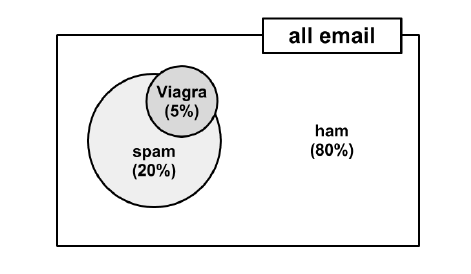top of page
Search

# Getting to Grips with - Naive Bayes (General Theory)Learning Classification Using Naïve Bayes

(Caveat: This Blog post is adapted from Chapter 4 of 'Machine Learning in R' by Lantz (2013)

In this Blog post we will explain the probabilistic learning algorithm,

Naive Bayes, which is widely used in ‘Big Data’ projects in the current climate although it has been used for predicting the weather, among other things, for quite some time. Naïve Bayes also uses principles of probability for classification. Just as meteorologists forecast weather, naive Bayes uses data about prior events to estimate the probability of future events. For instance, a common application of naive Bayes uses the frequency of words in past junk email messages to identify new junk mail and we will be guiding you through just such an example later.

Typically, Bayesian methods utilize all available evidence to subtly change the predictions.

Bayesian probability theory is rooted in the idea that the estimated likelihood of an event should be based on the evidence at hand. Key words are: ‘Events’ and ‘Trial’. Events are possible outcomes while a trial is a single opportunity for the event to occur, such as a coin flip or day’s weather.

Again, we will be talking the reader through an abbreviated step by step example as suggested by Lantz (2013) in the next Blog.

“Classifiers based on Bayesian methods utilize training data to calculate an observed probability of each class based on feature values. When the classifier is used later, on unlabelled data, it uses the observed probabilities to predict the most likely class for the new features” Lantz, (2013).

Lantz, (2013) goes on to explain that; “Typically, Bayesian classifiers are best applied to problems in which the information from numerous attributes should be considered simultaneously in order to estimate the probability of an outcome. While many algorithms ignore features that have weak effects, Bayesian methods utilize all available evidence to subtly change the predictions. If a large number of features, have relatively minor effects, taken together their combined impact could be quite large.”

Typical problems tackled using Bayseian methods might be:

• Text classification, such as junk email (spam) filtering, author identification, or topic categorization

• Intrusion detection or anomaly detection in computer networks

• Diagnosing medical conditions, when given a set of observed symptoms

Understanding Probability

The probability of an event can be estimated from observed data by dividing the number of trials in which an event occurred by the total number of trials. P(a)=0.20 for example (Probability of an event ‘A’ being 20%).

If the trial, mentioned above, has only two outcomes that cannot occur simultaneously then knowing the probability of one outcome reveals the other. Like heads or tails on a coin.

It is often helpful to imagine probability as a two-dimensional space that is partitioned into event probabilities for events.

In the following diagram, the rectangle represents the set of all possible outcomes for an email message. The circle represents the probability that the message is spam. The remaining 80 percent represents the messages that are not spaProbability_Illustrated

Joint Probability

Often, we are interested in monitoring several non-mutually exclusive events for the same trial. If some events occur with the event of interest, we may be able to use them to make predictions. Consider, for instance, a second event based on the outcome that the email message contains the word Viagra. For most people, this word is only likely to appear in a spam message; its presence in a message is therefore a very strong piece of evidence that the email is spam. The preceding diagram, updated for this second event, might appear as shown in the following diagram:Probability_with_Key_Word_Illustrated

But not all Viagra mentions in e-mails are spam and not all e-mails contain the word Viagra hence we should view these other possibilities, as seen in the Venn diagram below, which serves as a reminder to allocate probability to all possible combinations of events:Probability_with_other_outcomes_Illustrated

We might know that 20% of e-mail messages were without the word Viagra (left circle) and 5% appearing in ham (None Spam) on the right so we have to work out the overlap of P(Spam) and P(Viagra) occurring. This depends on how the joint probability of one event occurring and how it relates to the other event occurring. If the two events are totally unrelated then they are independent variables. Dependent events form the basis of predictive modelling, like the presence of clouds on a rainy day or the word Viagra predicting Spam e-mail.